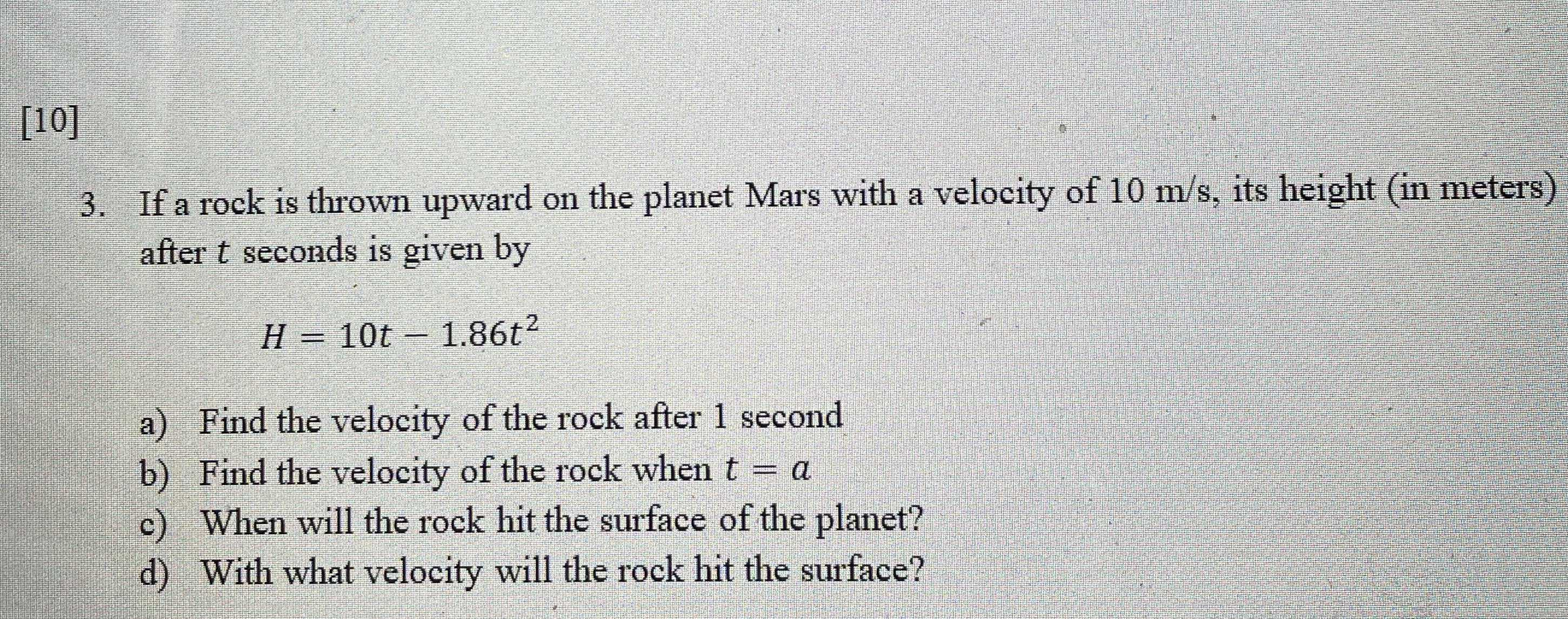### ¿Todavía tienes preguntas de matemáticas?

Pregunte a nuestros tutores expertos
Algebra
Pregunta3. If a rock is thrown upward on the planet Mars with a velocity of $$10 m / s$$ , its height (in meters) after $$t$$ seconds is given by

$$H = 10 t - 1.86 t ^ { 2 }$$

a) Find the velocity of the rock after $$1$$ second b) Find the velocity of the rock when $$t = a$$ c) When will the rock hit the surface of the planet? d) With what velocity will the rock hit the surface?